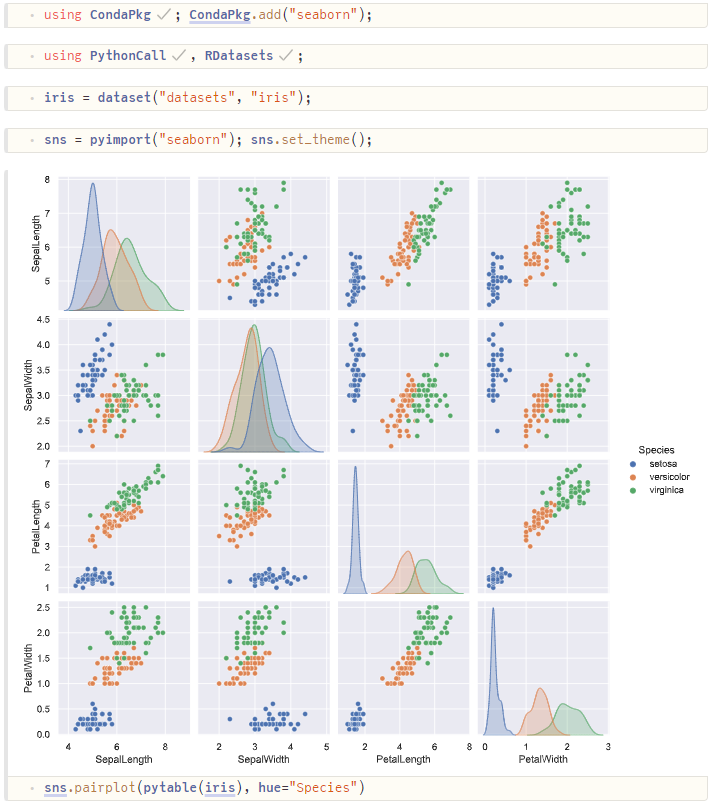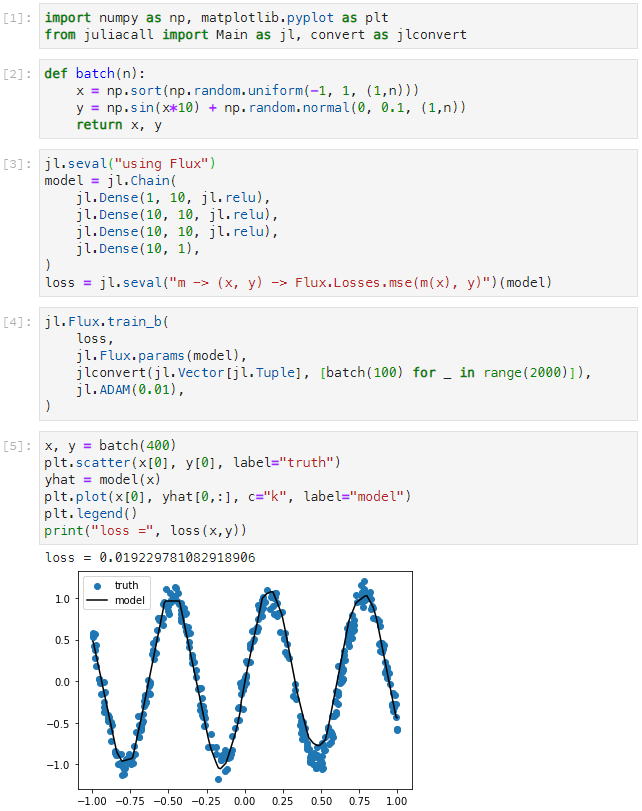PythonCall.jlBringing Python® and Julia together in seamless harmony:

• Call Python code from Julia and Julia code from Python via a symmetric interface.
• Simple syntax, so the Python code looks like Python and the Julia code looks like Julia.
• Intuitive and flexible conversions between Julia and Python: anything can be converted, you are in control.
• Fast non-copying conversion of numeric arrays in either direction: modify Python arrays (e.g. bytes, array.array, numpy.ndarray) from Julia or Julia arrays from Python.
• Helpful wrappers: interpret Python sequences, dictionaries, arrays, dataframes and IO streams as their Julia couterparts, and vice versa.
• Beautiful stack-traces.
• Works anywhere: tested on Windows, MacOS and Linux, 32- and 64-bit, Julia 1.0 upwards and Python 3.5 upwards.

To get started, read the documentation.

Example 1: Calling Python from Julia

In this example, we use PythonCall.jl from an IJulia notebook to inspect the Iris dataset:

• We load the Iris dataset as a Julia DataFrame using RDatasets.jl.
• We use pypandasdataframe(df) to convert it to a Python pandas.DataFrame.
• We use the Python package seaborn to produce a pair-plot, which is automatically displayed.Example 2: Calling Julia from Python

In this example we use the Python module juliacall from an IPython notebook to train a simple neural network:

• We generate some random training data using Python's numpy.
• We construct and train a neural network model using Julia's Flux.
• We plot some sample output from the model using Python's matplotlib.pyplot.